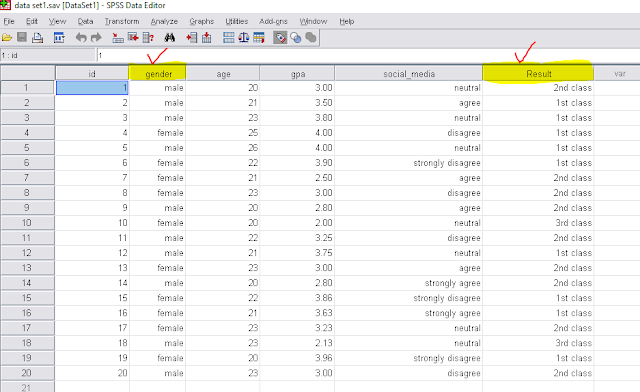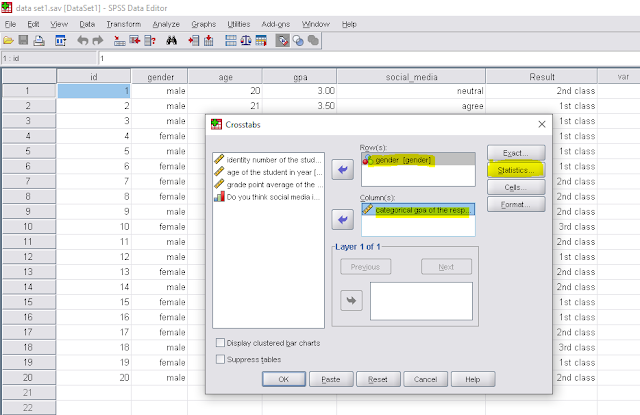# Bivariate analysis| How to analyze data using spss (part-10)Bivariate Analysis

### What is bivariate analysis?

Bivariate analysis is the analysis of two variable and find their association. For bivariate analysis we mainly use crosstabs and to show the association we use chi-square test. in chi-square table, we interpret the p-values. P-values interpretation is following-
• A small p-value (typically ≤ 0.05) indicates strong evidence against the null hypothesis, so we reject the null hypothesis.
• A large p-value (> 0.05) indicates weak evidence against the null hypothesis, so we fail to reject the null hypothesis.
• p-values very close to the cutoff (0.05) are considered to be marginal.

For bivariate analysis in spss we use the following data set and conduct bivariate analysis for the two highlighted variable (gender and result) and we also fond their association.Data set 1.First we have to go to-- Analyze →→→Descriptive statistics →→→→CrosstabsCrosstabs 2.Now we can see two box named Row and Column and we have to enter one variable into Row box and another into column box and click on statistics.Row and column box
3. In statistics we have to select chi-square and click on continue.Chi-square

4. Finally we have to click on OK and then we the see the output window.click on OK

OUTPUT

### Interpretation:

To interpret the chi square value we have build a hypothesis for association test. Let,
H0: There is no association between gender and result, vs

H1: H0 is not true.

Here we have a p-value 0.895 which is greater than 0.05. we we can not reject our null hypothesis. That means our null hypothesis is accepted and there is no association between gender and result.
• A small p-value (typically ≤ 0.05) indicates strong evidence against the null hypothesis, so we reject the null hypothesis.
• A large p-value (> 0.05) indicates weak evidence against the null hypothesis, so we fail to reject the null hypothesis.
• p-values very close to the cutoff (0.05) are considered to be marginal.

How to analyze data using SPSS (for beginners)-part 1
How to analyze data using SPSS (part-2), how to input data in SPSS
How to analyze data using SPSS (part-3), how to import data in SPSS from excel file
How to analyze data using SPSS (part-4), how to sort data in SPSS
How to analyze data using SPSS ( part-5) how to merge file in SPSS?
How to analyze data using SPSS ( part-6), how to merge file in SPSS
How to analyze data using SPSS (part-7), Finding missing values, Replacing missing values, Coding missing values in SPSS
How to analyze data using SPSS (part-8), variable transformation, Recoding variables in spss
Bivariate analysis| How to analyze data using spss (part-10)
Normality check| how to analyze data using spss (part-11)